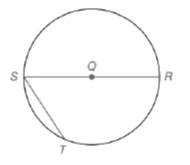Chapter 6.1, Problem 10E### Elementary Geometry for College St...

6th Edition
Daniel C. Alexander + 1 other
ISBN: 9781285195698

#### Solutions

Chapter
Section### Elementary Geometry for College St...

6th Edition
Daniel C. Alexander + 1 other
ISBN: 9781285195698
Textbook Problem
3 views

# Given: S T = 1 2 ( S R )  in  ⊙ Q   S R ¯  is a diameterFind: a) m S T ⌢ b) m T R ⌢ c) m S T R ⌢ d) m ∠ S (HINT: Dra Q T ¯ . )

To determine

a)

To calculate:

The mST from the provided figure.

Explanation

Given:

In a circle Q, ST=12(SR) where SR¯ is the diameter.

Theorem used:

The measure of an inscribed angle of a circle is one-half the measure of its intercepted arc.

An angle inscribed in a semicircle is a right angle.

Calculation:

From the figure we see that STR is a semicircle. Join TR¯ as shown below

We know that the angle inscribed in a semicircle is a right angle. Therefore STR=90.

Given that ST=12(SR).

In a right triangle STR, the hypotenuse is twice as longer than the shorter leg.

Hence the angles in right triangle STR are 30,60,90

To determine

b)

To calculate:

The mTR from the provided figure.

To determine

c)

To calculate:

The mSTR from the provided figure.

To determine

d)

To calculate:

The mS from the provided figure.

### Still sussing out bartleby?

Check out a sample textbook solution.

See a sample solution

#### The Solution to Your Study Problems

Bartleby provides explanations to thousands of textbook problems written by our experts, many with advanced degrees!

Get Started

#### In Exercises 2124, factor each expression completely. 23. 16 x2

Applied Calculus for the Managerial, Life, and Social Sciences: A Brief Approach

#### Differentiate the function. y=x+xx2

Single Variable Calculus: Early Transcendentals, Volume I

#### Evaluate the expression sin Exercises 116. (23)2

Finite Mathematics and Applied Calculus (MindTap Course List)

#### True or False: n=1(1)nnn+3 converges.

Study Guide for Stewart's Single Variable Calculus: Early Transcendentals, 8th